### Home > MC2 > Chapter 8 > Lesson 8.2.5 > Problem8-78

8-78.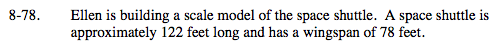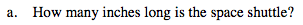Recall that 12 inches is equivalent to 1 foot.

$\frac{\text{1 ft}}{\text{12 inches}} \left( \frac{?}{?} \right) = \frac{\text{122 ft}}{x\text{ inches}}$

1464 inches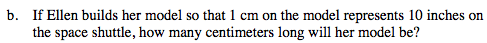Try using the same method presented in (a).

$\frac{\text{1 cm}}{\text{10 inches}} \left( \frac{?}{?} \right) = \frac{\text{x cm}}{\text{1464 inches}}$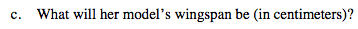Begin by finding the length of the wingspan in inches, as presented in (a).
Then find the length the model needs to be in centimeters, as in (b).

93.6 cm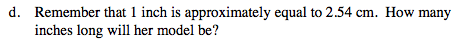$\frac{\text{1 inch}}{\text{2.54 cm}} \left( \frac{?}{?} \right) = \frac{x\text{ inches}}{146.4}$